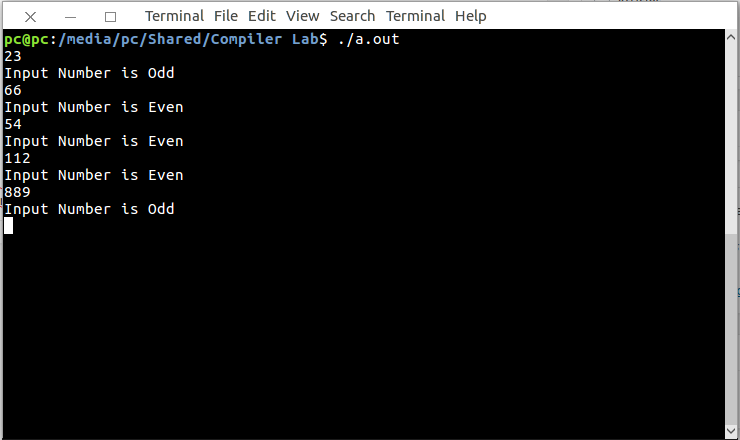Open in App
Not now

# Lex program to check whether input number is odd or even

• Last Updated : 30 Apr, 2019

Lex is a computer program that generates lexical analyzers. Lex reads an input stream specifying the lexical analyzer and outputs source code implementing the lexer in the C programming language.

The commands for executing the lex program are:

```lex abc.l (abc is the file name)
gcc lex.yy.c -ll
./a.out
```

Let’s see lex program to check whether input number is odd or even.

Examples:

```Input :
22
Output :
Input Number is Even

Input :
53
Output :
Input Number is odd
```

Below is the implementation:

 `/% Lex Program to check whether  ` `      ``- input number is odd or even. %/ ` ` `  `% { ` `   ``int` `i; ` `% } ` ` `  `%% ` `[0-9]+ {i = ``atoi``(yytext);  ` ` `  `if``(i%2==0)  ` ` ``printf``(``"Input Number is Even"``);  ` ` `  `else`  ` ``printf``(``"Input Number is Odd"``); ` `}; ` ` `  `%% ` ` ``int` `main() ` `  ``{ ` `    ``yylex(); ` `    ``return` `1; ` `  ``} `

Output:My Personal Notes arrow_drop_up
Related Articles